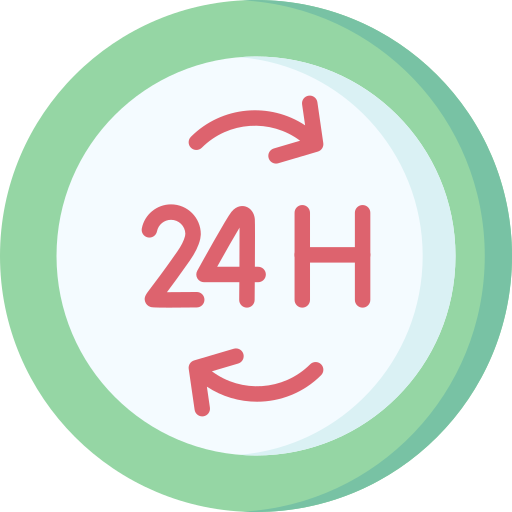# Tips And Tricks And Shortcuts For Clocks

## Shortcuts For Clocks In Aptitude

Here on this page you will get some easy Tips and Tricks and Shortcut For Clocks  based Questions which will efficiently help in the competitive exam. Go through this page for proper understanding.### Tips & Tricks for Clocks to solve problems easily

Below are some easy tips and tricks for you on problems based on clocks which efficiently help in the competitive exam . It is a very important topic for the recruitment drive.

• Speed of the minute hand = 6° per minute.
• Speed of the hour hand = 0.5° per minute.
• Relative Speed between hour and minute hand. The relative speed of the minute hand is 6 – 0.5 dpm = 5.5 dpm. Where dpm =  dial per minute.
• Angle between Hands: To find the angle between the hour and minute hands, use the formula: |(30 x Hour – 5.5 x Minutes)|.
• Acute Angle: To find the acute angle between the hands, subtract the smaller angle from the larger angle, and take the absolute value.

• Obtuse Angle: To find the obtuse angle between the hands, subtract 360 degrees from the acute angle.

• Shortcut for 180 degrees: Whenever the minute hand points to 6 and the hour hand is at any number (1 to 11), they form a 180-degree angle.

• Shortcut for 0 degrees: Whenever the minute hand and hour hand overlap (coincide), the angle between them is 0 degrees.

### Type 1:  Shortcuts on Clocks.

Question 1. What is the angle between the minute hand and the hour hand when the time is 5:30.

Options:

A.15 °

B. 10°

C. 20°

D. None of the above

Solution:

Angle covered by the hour hand (H) at 5:30:
Since the hour hand moves 30 degrees for each hour on the clock, at 5:30, it covers 5.5 hours (5 hours and 30 minutes). So, the angle covered by the hour hand is H = 5.5 x 30 = 165°

Angle covered by the minute hand (M) at 5:30:
The minute hand moves 6 degrees for each minute on the clock. At 5:30, it covers 30 minutes. So, the angle covered by the minute hand is M = 30 x 6 = 180°

Calculating the absolute difference between the two angles,

Angle between the hour and minute hands (A) = H – M = 165 – 180= 15°

Correct Option: A

Question 2. At what time between 4 o’clock and 5 o’clock will the minute hand and the hour hand of the clock form a 90 ° angle?

Solution:

Let’s assume the time is x minutes past 4 o’clock.

Angle covered by the hour hand in x minutes $= \frac{30}{60}\times x = \frac{x}{2}$°.

Angle covered by the minute hand in x minutes
$=\frac{360}{60}\times x=6x$°.

For 90° angle between the hands, the difference between their angles should be 90°.

So, 6x – x/2 = 90

12x – x = 180

11x = 180

x = 180/11 ≈ 16.36 minutes

Therefore, the hands form a 90° angle approximately 16.36 minutes past 4 o’clock.

### Type 2: Shortcuts to Solve Clocks

Question 1. Calculate the time between 7 and 8 o’clock when the hands of a clock are in the same straight line but are not together?

Options:

A. 7:05(5/11 ) o’clock

B. 7:50 (1/13) o’clock

C. 7:20 o’clock

D. Cannot be determined

Solution:     Tip: The short formula to calculate the time when the angle is given is,

Angle = 30 (hours) – 5.5 (minutes)

Using the above formula, we get

180 = 30 (hours) – 11/2 (minutes)

180 =  30 (7) – 11/2 (minutes)

11/2 minutes = 210-180

Minutes = 30*2/11

= 5( 1/5)

Time = 7:05(5/11) o’clock

Correct Option: A

Question 2.What is the acute angle between the minute hand and the hour hand of a clock at 4:15?

Solution:

Angle covered by the hour hand at 4:15

$30\times Hour + (\frac{Minutes}{2})$

$30\times 4 + \frac{15}{2}=120+7.5=127.5$

Angle covered by the minute hand at 4:15 = $\frac{360}{60}\times Minutes$

$\frac{360}{60}\times15=90$

To find the acute angle between the hands: Acute angle = 127.5 – 90 = 37.5

Therefore, at 4:15, the acute angle between the minute hand and the hour hand is 37.5 degrees.

### Related Banners

Get PrepInsta Prime & get Access to all 200+ courses offered by PrepInsta in One Subscription

## Get over 200+ course One Subscription

Courses like AI/ML, Cloud Computing, Ethical Hacking, C, C++, Java, Python, DSA (All Languages), Competitive Coding (All Languages), TCS, Infosys, Wipro, Amazon, DBMS, SQL and others